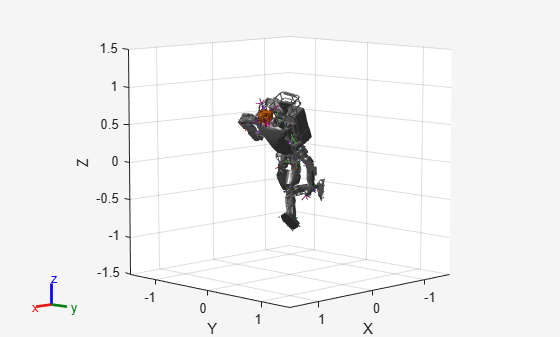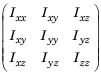# massMatrix

Joint-space mass matrix

## Syntax

``H = massMatrix(robot)``
``H = massMatrix(robot,configuration)``

## Description

````H = massMatrix(robot)` returns the joint-space mass matrix of the home configuration of a robot.```

example

````H = massMatrix(robot,configuration)` returns the mass matrix for a specified robot configuration.```

## Examples

collapse all

Load a model of the Boston Dynamics Atlas® humanoid robot from the Robotics System Toolbox™ `loadrobot`, returned as a `rigidBodyTree` object. Ensure that the data format is set to "`row"`. For all dynamics calculations, the data format must be either "`row"` or "`column"`.

`atlas = loadrobot("atlas", DataFormat="row", Gravity=[0 0 -9.81]);`

Generate a random configuration for `atlas`.

`q = randomConfiguration(atlas);`

Get the mass matrix at configuration `q`.

`H = massMatrix(atlas,q)`
```H = 30×30 5.9158 -1.2431 0.6367 0.4879 -0.5435 0.1373 0.1765 -0.0007 -0.0072 -0.0003 -0.0001 0.9609 0.3663 -0.0521 -0.3232 -0.0269 0.0011 0.0001 0 0 0 0 0 0 0 0 0 0 0 0 -1.2431 20.9002 0.5109 -0.5926 0.8585 0.1457 -0.0027 -0.0003 0.0018 -0.0002 -0.0018 -0.0413 0.2023 0.0525 0.1088 0.0236 0.0108 -0.0005 0 0 0 0 0 0 0 0 0 0 0 0 0.6367 0.5109 19.4204 0.2148 0.6959 -0.3081 -0.2676 0.0004 0.0074 0.0004 0.0000 0.0330 0.9184 0.0043 -0.3224 -0.0142 0.0250 0.0002 0 0 0 0 0 0 0 0 0 0 0 0 0.4879 -0.5926 0.2148 0.2945 -0.0589 0.0975 0.0317 -0.0013 0.0032 -0.0003 0 0 0 0 0 0 0 0 0 0 0 0 0 0 0 0 0 0 0 0 -0.5435 0.8585 0.6959 -0.0589 0.5987 -0.0512 0.0887 0.0014 -0.0112 0.0002 0 0 0 0 0 0 0 0 0 0 0 0 0 0 0 0 0 0 0 0 0.1373 0.1457 -0.3081 0.0975 -0.0512 0.2033 -0.0008 -0.0010 0.0090 -0.0004 0 0 0 0 0 0 0 0 0 0 0 0 0 0 0 0 0 0 0 0 0.1765 -0.0027 -0.2676 0.0317 0.0887 -0.0008 0.3040 0.0009 -0.0276 -0.0000 0 0 0 0 0 0 0 0 0 0 0 0 0 0 0 0 0 0 0 0 -0.0007 -0.0003 0.0004 -0.0013 0.0014 -0.0010 0.0009 0.0038 -0.0000 0.0005 0 0 0 0 0 0 0 0 0 0 0 0 0 0 0 0 0 0 0 0 -0.0072 0.0018 0.0074 0.0032 -0.0112 0.0090 -0.0276 -0.0000 0.0070 -0.0000 0 0 0 0 0 0 0 0 0 0 0 0 0 0 0 0 0 0 0 0 -0.0003 -0.0002 0.0004 -0.0003 0.0002 -0.0004 -0.0000 0.0005 -0.0000 0.0005 0 0 0 0 0 0 0 0 0 0 0 0 0 0 0 0 0 0 0 0 ⋮ ```
`show(atlas,q);`## Input Arguments

collapse all

Robot model, specified as a `rigidBodyTree` object. To use the `massMatrix` function, set the `DataFormat` property to either `'row'` or `'column'`.

Robot configuration, specified as a vector with positions for all nonfixed joints in the robot model. You can generate a configuration using `homeConfiguration(robot)`, `randomConfiguration(robot)`, or by specifying your own joint positions. To use the vector form of `configuration`, set the `DataFormat` property for the `robot` to either `'row'` or `'column'`.

## Output Arguments

collapse all

Mass matrix of the robot, returned as a positive-definite symmetric matrix with size n-by-n, where n is the velocity degrees of freedom of the robot.

collapse all

### Dynamics Properties

When working with robot dynamics, specify the information for individual bodies of your manipulator robot using these properties of the `rigidBody` objects:

• `Mass` — Mass of the rigid body in kilograms.

• `CenterOfMass` — Center of mass position of the rigid body, specified as a vector of the form `[x y z]`. The vector describes the location of the center of mass of the rigid body, relative to the body frame, in meters. The `centerOfMass` object function uses these rigid body property values when computing the center of mass of a robot.

• `Inertia` — Inertia of the rigid body, specified as a vector of the form `[Ixx Iyy Izz Iyz Ixz Ixy]`. The vector is relative to the body frame in kilogram square meters. The inertia tensor is a positive definite matrix of the form:The first three elements of the `Inertia` vector are the moment of inertia, which are the diagonal elements of the inertia tensor. The last three elements are the product of inertia, which are the off-diagonal elements of the inertia tensor.

For information related to the entire manipulator robot model, specify these `rigidBodyTree` object properties:

• `Gravity` — Gravitational acceleration experienced by the robot, specified as an `[x y z]` vector in m/s2. By default, there is no gravitational acceleration.

• `DataFormat` — The input and output data format for the kinematics and dynamics functions, specified as `"struct"`, `"row"`, or `"column"`.

### Dynamics Equations

Manipulator rigid body dynamics are governed by this equation:

`$\frac{d}{dt}\left[\begin{array}{c}q\\ \stackrel{˙}{q}\end{array}\right]=\left[\begin{array}{c}\stackrel{˙}{q}\\ M{\left(q\right)}^{-1}\left(-C\left(q,\stackrel{˙}{q}\right)\stackrel{˙}{q}-G\left(q\right)-J{\left(q\right)}^{T}{F}_{Ext}+\tau \right)\end{array}\right]$`

also written as:

`$M\left(q\right)\stackrel{¨}{q}=-C\left(q,\stackrel{˙}{q}\right)\stackrel{˙}{q}-G\left(q\right)-J{\left(q\right)}^{T}{F}_{Ext}+\tau$`

where:

• $M\left(q\right)$ — is a joint-space mass matrix based on the current robot configuration. Calculate this matrix by using the `massMatrix` object function.

• $C\left(q,\stackrel{˙}{q}\right)$ — are the Coriolis terms, which are multiplied by $\stackrel{˙}{q}$ to calculate the velocity product. Calculate the velocity product by using by the `velocityProduct` object function.

• $G\left(q\right)$ — is the gravity torques and forces required for all joints to maintain their positions in the specified gravity `Gravity`. Calculate the gravity torque by using the `gravityTorque` object function.

• $J\left(q\right)$ — is the geometric Jacobian for the specified joint configuration. Calculate the geometric Jacobian by using the `geometricJacobian` object function.

• ${F}_{Ext}$ — is a matrix of the external forces applied to the rigid body. Generate external forces by using the `externalForce` object function.

• $\tau$ — are the joint torques and forces applied directly as a vector to each joint.

• $q,\stackrel{˙}{q},\stackrel{¨}{q}$ — are the joint configuration, joint velocities, and joint accelerations, respectively, as individual vectors. For revolute joints, specify values in radians, rad/s, and rad/s2, respectively. For prismatic joints, specify in meters, m/s, and m/s2.

To compute the dynamics directly, use the `forwardDynamics` object function. The function calculates the joint accelerations for the specified combinations of the above inputs.

To achieve a certain set of motions, use the `inverseDynamics` object function. The function calculates the joint torques required to achieve the specified configuration, velocities, accelerations, and external forces.

## Version History

Introduced in R2017a